# RPF 5 Jan 2019

Instructions

For the following questions answer them individually

Question 91

# Each face of the dice is painted with Red, Green, Gray, Blue, Brown and Orange as shown in the given figure. What colour is painted on the face opposite to Orange colour?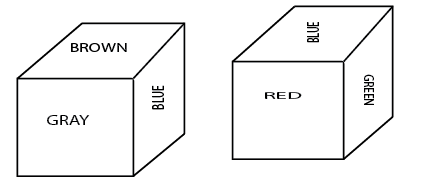Question 92

# In this question, relationship between different elements is shown in the statement. This statement is followed by two conclusions:Statements: K = I < N ≤ D < E > R > AConclusion:i) K < Eii) E > AChoose the appropriate one from the following options(A)Only conclusion i follows.(B) Only conclusion ii follows.(C) Either conclusion i or ii follows.(D) Neither conclusion i nor ii follows.(E) Both conclusion i and ii follow.Question 93

# Choose the correct alternative which will complete the image pattern of the given image.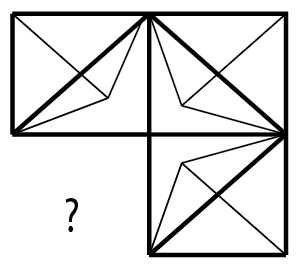Question 94

# If a mirror is placed on the shaded line, then which of the following options is the right image of the given figure?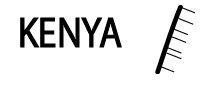Question 95

# Choose the correct water image of the given question from the given options.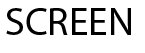Question 96

# Replace the question mark with an option that follows the same logic applied in the first pairPathology:Diseases::Paleontology: ??Question 97

# How many straight lines are there in the given figure?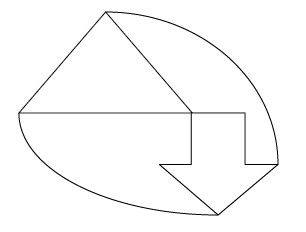Question 98

# Which one of the alternative will be the next figure from the given alternatives for the given series?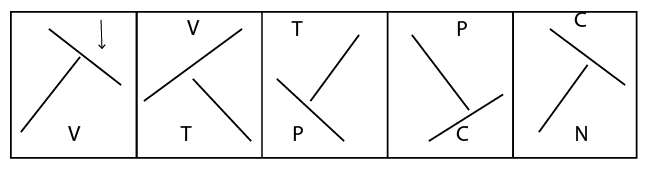Question 99

# How many Right angled triangles can be formed from the given figure?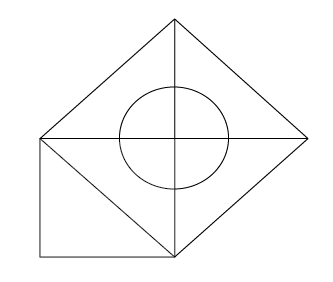Question 100

# Select the correct alternative from the following options which can form a complete square (3 out of 5 images given below) :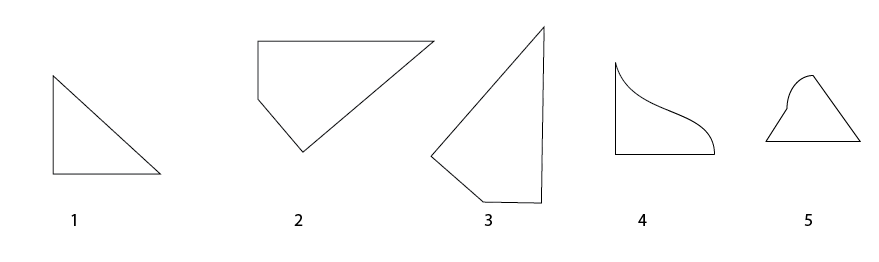OR